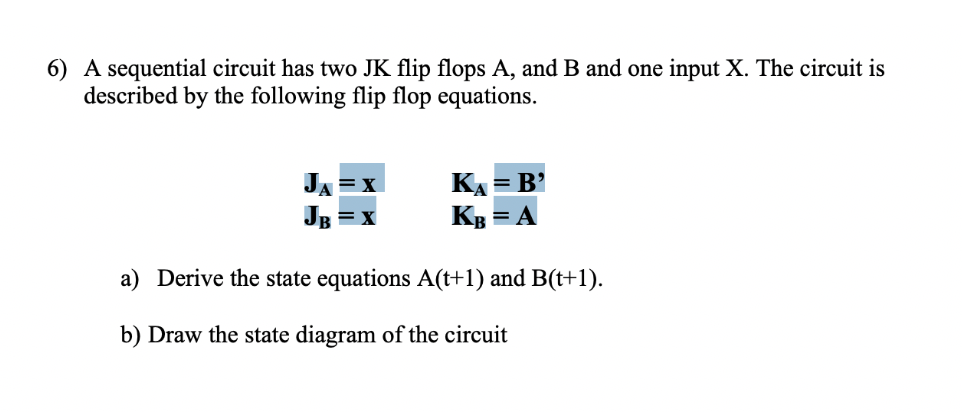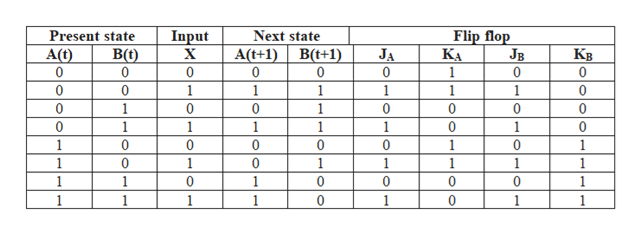# 6) A sequential circuit has two JK flip flops A, and B and one input X. The circuit isdescribed by the following flip flop equationsКА 3 В'КвJA= Aa) Derive the state equations A(t+1) and B(t+1)b) Draw the state diagram of the circuit

Question
420 viewshelp_outlineImage Transcriptionclose6) A sequential circuit has two JK flip flops A, and B and one input X. The circuit is described by the following flip flop equations КА 3 В' Кв JA = A a) Derive the state equations A(t+1) and B(t+1) b) Draw the state diagram of the circuit fullscreen
check_circle

Step 1

a)

State equation:

Step 2

Characteristic equation:

Step 3

Excitation table:

...help_outlineImage TranscriptioncloseFlip flop Jв 0 Present state Input х Next state В(О) 0 B(t+1) 0 JA КА Кв A(t) 0 A(t+1) 0 0 1 0 0 0 1 1 1 1 1 0 0 0 0 1 0 0 1 1 1 1 1 1 0 0 0 0 0 0 0 1 1 1 1 0 1 0 1 1 1 1 1 1 1 1 0 0 1 1 1 1 0 1 0 1 fullscreen

### Want to see the full answer?

See Solution

#### Want to see this answer and more?

Solutions are written by subject experts who are available 24/7. Questions are typically answered within 1 hour.*

See Solution
*Response times may vary by subject and question.
Tagged in

### Computer Science